## Friday, January 03, 2020

### The Real Butterfly Effect

If a butterfly flaps its wings in China today, it may cause a tornado in America next week. Most of you will be familiar with this “Butterfly Effect” that is frequently used to illustrate a typical behavior of chaotic systems: Even smallest disturbances can grow and have big consequences.

The name “Butterfly Effect” was popularized by James Gleick in his 1987 book “Chaos” and is usually attributed to the meteorologist Edward Lorenz. But I recently learned that this is not what Lorenz actually meant by Butterfly Effect.

I learned this from a paper by Tim Palmer, Andreas Döring, and Gregory Seregin called “The Real Butterfly Effect” and that led me to dig up Lorenz’ original paper from 1969.

Lorenz, in this paper, does not write about butterfly wings. He instead refers to a sea gull’s wings, but then attributes that to a meteorologist whose name he can’t recall. The reference to a butterfly seems to have come from a talk that Lorenz gave in 1972, which was titled “Does the Flap of a Butterfly’s Wings in Brazil set off a Tornado in Texas?”

The title of this talk was actually suggested by the session chair, a meteorologist by name Phil Merilees. In any case, it was the butterfly that stuck instead of the sea gull. And what was the butterfly talk about? It was a summary of Lorentz 1969 paper. So what’s in that paper?

In that paper, Lorenz made a much stronger claim than that a chaotic system is sensitive to the initial conditions. The usual butterfly effect says that any small inaccuracy in the knowledge that you have about the initial state of the system will eventually blow up and make a large difference. But if you did precisely know the initial state, then you could precisely predict the outcome, and if only you had good enough data you could make predictions as far ahead as you like. It’s chaos, alright, but it’s still deterministic.

Now, in the 1969 paper, Lorenz looks at a system that has an even worse behavior. He talks about weather, so the system he considers is the Earth, but that doesn’t really matter, it could be anything. He says, let us divide up the system into pieces of equal size. In each piece we put a detector that makes a measurement of some quantity. That quantity is what you need as input to make a prediction. Say, air pressure and temperature. He further assumes that these measurements are arbitrarily accurate. Clearly unrealistic, but that’s just to make a point.

How well can you make predictions using the data from your measurements? You have data on that finite grid. But that does not mean you can generally make a good prediction on the scale of that grid, because errors will creep into your prediction from scales smaller than the grid. You expect that to happen of course because that’s chaos; the non-linearity couples all the different scales together and the error on the small scales doesn’t stay on the small scales.

But you can try to combat this error by making the grid smaller and putting in more measurement devices. For example, Lorenz says, if you have a typical grid of some thousand kilometers, you can make a prediction that’s good for, say, 5 days. After these 5 days, the errors from smaller distances screw you up. So then you go and decrease your grid length by a factor of two.

Now you have many more measurements and much more data. But, and here comes the important point: Lorenz says this may only increase the time for which you can make a good prediction by half of the original time. So now you have 5 days plus 2 and a half days. Then you can go and make your grid finer again. And again you will gain half of the time. So now you have 5 days plus 2 and half plus 1 and a quarter. And so on.

Most of you will know that if you sum up this series all the way to infinity it will converge to a finite value, in this case that’s 10 days. This means that even if you have an arbitrarily fine grid and you know the initial condition precisely, you will only be able to make predictions for a finite amount of time.

And this is the real butterfly effect. That a chaotic system may be deterministic and yet still be non-predictable beyond a finite amount of time .

This of course raises the question whether there actually is any system that has such properties. There are differential equations which have such a behavior. But whether the real butterfly effect occurs for any equation that describes nature is unclear. The Navier-Stokes equation, which Lorenz was talking about may or may not suffer from the “real” butterfly effect. No one knows. This is presently one of the big unsolved problems in mathematics.

However, the Navier-Stokes equation, and really any other equation for macroscopic systems, is strictly speaking only an approximation. On the most fundamental level it’s all particle physics and, ultimately, quantum mechanics. And the equations of quantum mechanics do not have butterfly effects because they are linear. Then again, no one would use quantum mechanics to predict the weather, so that’s a rather theoretical answer.

The brief summary is that even in a deterministic system predictions may only be possible for a finite amount of time and that is what Lorenz really meant by “Butterfly Effect.”

1.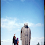With regard to quantum mechanics and chaos, John Barrow gave an example: Imagine that you can determine the position and momentum of a billiard ball to the precision allowed by the uncertainty relation. After how many collisions is the average ball position larger than the size of the table? I don't remember the answer, but I believe less than a dozen.

1.Well, you should really catch-up and have a look at the work of Katsuhiro Nakamura, if you're to have the least idea. Quantum and chaos are incompatible according to our present understanding. What we need is a physical theory that is compatible with mathematical chaos, too!

2.So someone suggested a talk which included the butterfly effect but the talk was primarily about something else, but that doesn't mean that the topic of the talk is the real butterfly effect.

The discovery is interesting. He printed out the values of variables, then re-started the calculation with those as the input, and noticed that it evolved differently than the original calculation, and put it down to the roundoff errors in the written out output.

3.Yes, and the other side of the coin is that, by virtue of its mixing properties, statistical properties arise from the flow. Indeed you cannot predict individual trajectories, but you can obtain statistical predictions for an ensemble thereof.

4."Undecidability, Uncomputability, and Unpredictability Essay Contest"

5.Nice blogpost! :)

Re: "Then again, no one would use quantum mechanics to predict the weather" why not? In practical terms (e.g. computing resource limitations), what is the largest "weather" system that can be simulated, using QM? Similar question: at what scale does the Navier-Stokes equation cease to be a good approximation to the behavior of fluids?

1.JeanTate, the issue is not the "practical terms", but the usual issue of classical emergence. If you were to solely evolve the Schrodinger equation, you'd never get anything measurable, because at some point you need to apply the Born rule to evaluate the measurement outcomes seen by a classical detector. So, "using quantum mechanics to simulate a classical system" is a red herring until the mystery of the Born rule emergence is resolved... Hopefully some day soon.

2.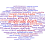To simulate fluids with QM: from what I know, true first-principle models are limited to something like O(100) atoms or less. Molecular dynamics is hard to simulate fluids with, and it's a classical/phenomenological approach caricaturing the real QM interactions, but can get close to things on the scale of cubic centimeters with some tricks if I recall (and on massive computers). Remember that Avogodro's number is on the order of 10^26, and so you have at least this many ordinary differential equations (in the classical approximation), and the QM approach is substantially harder. At every level there are tricks and approximations, so that the "macroscale" and "ground truth" vary as you move along scales. In my area of biophysics, we usually consider molecular dynamics as ground truth, but as you can see that is just an approximation.

The second question is related to the Knudsen number of the fluid, and very loosely speaking, implies that the continuum models of fluids break down somewhere close to the micron scale. Of course we don't know how important small errors from this breakdown are on larger scales, and some turbulence research suggests this as important, but as far as I'm aware it's an open question.

3.Avogadro's number is 10^23, but that doesn't change much.

4.Oof that's definitely a big error. But yeah, still far too large of a system to solve in practice, though there are many good approximations in different contexts.

6.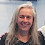I can't find the diagram that I came across when I was first introduced to Chaos theory in the 1980s.

IIRC one could plot the variables in 3D, and from one perspective it looked like discontinuous break, but from the other you could see the factors that were causing the break in the expected outcome.

I used it for game theory and manipulation of random outcomes. Fascinating stuff.

7."However, the Navier-Stokes equation, and really any other equation for macroscopic systems, is strictly speaking only an approximation. "

I would say that all equations are approximations. In what sense could it be otherwise? What would it even mean to have "true" equations? How would it be knowable if we have "true" equations and not just approximations?

Of course some macroscopic things one can explain by microscopic models. But these microscopic models are still approximations.

1.Jukka,

The point is that in some cases we already know that they are only approximations, such as for the Navier-Stokes equations. Quantum mechanics, on the other hand, is currently the most fundamental description of nature that we have. If it is an approximation to something else, we don't know what that something else is.

2."Quantum mechanics, on the other hand, is currently the most fundamental description of nature that we have. If it is an approximation to something else, we don't know what that something else is."

But that will always be the case. Currently we know something, but in the future we may have a different point of view.

I think the word model is convenient here. There are different models of "reality", and different models are convenient in different contexts. In this sense one could say that it is even natural to have incompatible models, like quantum mechanics and relativity, because they are different models with different purposes and different points of view.

Let's take a concrete example. In general relativity the ambient space is considered as a smooth manifold. To me this is a model (or approximation if you prefer). Smooth manifolds (and differential geometry in general) are extremely useful, but this is just one way to view things.

The equations of physics are often PDE systems. Now there are many different ways to consider what are "acceptable" solutions of these systems. This is also a question of modeling. Does the "nature" accept only smooth solutions? (already in classical physics we know that the answer is no) But when we accept weak solutions there is no unique way to characterize solutions. There can be different kinds of solutions, depending how weak solutions you are willing to accept.

So in summary I think that models or approximations is all we can have. Evidently more unified theories would be nice, but they are still models or approximations.

8.The paper by Ball that you link to seems to be about blowup of solutions rather than the butterfly effect. Is your point that if the weather blows up in 10 days then it cannot be predicted for more than 10 days? Also, is it possible for a system to be unpredictable beyond some fixed horizon (say, 10 days) even if it doesn't experience any blowup?

1.No, as I said, no one knows whether the Navier-Stokes equation has such a behavior. The paper has examples for differential equations whose solutions can't be continued past a finite amount of time. That they "blow up" is kind of not the relevant point. The point is that they develop a singularity.

2.Unpredictability and blow up are a priori not related.

The word singularity has many meanings in different contexts. It is best to think about blow ups as some types of singularity. (note that in algebraic geometry there is a technical term "blow up" which is unrelated to the present topic)

If the solution becomes singular at some point in time then it may or may not be possible to continue the solution as a generalized solution for larger times. For example certain PDE (known as conservation laws) can have well defined discontinuous solutions (shocks, entropy solutions) after the classical solution ceases to exist.

The paper by Ball is very old. The analysis of various types of solutions/singularities to PDE is still an active area of research.

3.Nice clarification! I've always had problems with the usual interpretation, based on a point by Bengtssom in another post-- friction, dissipation of energy in many ways, damping factors. The same kind of stuff that makes a perpetual motion machine impossible. As usual I appreciate the effort you put into this post.

4.Sabine said - The paper has examples for differential equations whose solutions can't be continued past a finite amount of time. and before she said- And the equations of quantum mechanics do not have butterfly effects because they are linear. -And I wonder ; Is she trying to build a quantum space to describe a whole?

5.I mean, the disorder exists within an order, from the perspective of the order you can see the disorder; as in a dance choreography someone fails

6.I also think that Ball's paper is out of context.

7.It provides an example for exactly what I said it provides an example for. If you think this is "out of context" then you did not understand the context.

8.But it doesn't. It gives an example of a system where the solution doesn't exist for all times. It blows up in finite time. But you wrote that it was an example of the real butterfly effect, which is about predictability of a solution that does exist.

I suppose I still don't understand the context. Can you clarify?

9.If I give you an initial condition and you integrate it forward and the solution "blows up" after a finite amount of time, you can't make predictions beyond that.

10.If course not, but that is not what the butterfly effect is about.

9.That's a very special butterfly. How do the other butterflies feel about this? The original folklore idea (and incorrect) of this was tantamount to saying pushing pins in a doll may affect something somewhere.

1.Does not everything affect everything in the speed of light due to propagation of the forces?

Even pushing a pin :)

10.Isn't there a "theoretical" and a "practical" problem? Some nonlinear system calculations are explosively divergent but I see nothing there that - to go the whole enchilada - rules out superdeterminism.

11.On the other hand, wouldn't it be more reasonable to assume that the effect of the flap of a butterfly's wing will damp out, and only cause local effects. Isn't damping a more common phenomena than amplification? What about the cluster decompostion principle? In any way, since it is presumably completely impossible to predict or retrodict that a certain tornado somewhere far off were caused by a minor disturbance somewhere else, why is this interesting at all?

12.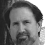Kronecker once said that God invented the integers, with everything else being man's invention. Theories proposing pure determinism for all time would have to be based on integers, since an uncertainty at the umpteenth decimal place of a non-integer would eventually lead to chaos.

13.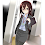The Lorenz equations emerge from a Galerkin spectral sequence with the Navier-Stokes (NS) equation for flow with gravity and a ground heat source. This is a model for atmospheric hydrodynamics. The Lorenz equations are the first order result.

The NS equation assumes a continuous fluid. That any fluid or gas is made of atoms is a cut-off in the model. If we could fine grain any simulation far enough we might run into a many body problem. This would lead to another depth, one that also has chaos.

Poincaré looked at systems with complex separatrices in phase space. A separatrix can have lots of filigree, which in a purely classical setting is an endless self-similar fractal. In phase space, however, the near loops can only bound an area equal to the Planck unit of action. We might the propose that decoherence of quantum waves is the ultimate dice roll that gives the butterfly wing flap.

14.So it is great opportunity to chec? if quantum uncertainty relations are for our knowledge, but not for real, arising Navier-Stokes equations should not grow various fluid excitations arising from uncertainty. On the other side of uncertainty relations are real, and moon doesn't exist if nobody is watching, classical chaotic, determinism systems should amplify this uncertainty and became in some ways, fundamental indeterministic.
Is there any way to check the difference?

15.For a boxer whose opponent floats like a butterfly and stings like a bee it is very difficult to predict his opponent's actions and their effects.

16.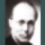So, what can we say about the exact connection of butterfly effect to the measurement problem?

17.In the study of financial market behaviour, the butterfly effect is invoked in support of the impredictability of financial turbulences: moments of frantic and extreme changes in the prices of the traded goods. The fractal nature of this phenomenon was addressed by B. Mandelbrot himself in his book "The (Mis)behaviour of Markets". This is a direct challenge to the "mainstream" definition of financial risk, which is based on the statistical properties of the ensemble. In this down to earth context, the "butterfly effect" disputes the validity the Gaussian model of the financial markets.

18.The question seems more mathematical than physical to me. For most differential equations that display instabilities, such instabilities are exponential (ie, take an infinite time to go to infinity). For some of them, the instability occurs in a finite time (finite-time blow-up). You can find mathematical examples of that, but it is not clear if the Navier-Stokes equation contain this type of solution.
In practice, the difference is not so important. As Philip pointed out, standard exponential instability is more than enough to randomize a system like the weather in a very short time (a few days).
PS: Contrarily to what is stated in the last paragraph, the fact that quantum mechanics does or does not display chaotic behavior has nothing to do with the linearity of the Schrodinger equation.

19.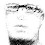If one were to actually take Max Tegmark's dictum* seriously - "Our challenge as physicists is to discover this elegant way [of doing without infinities] and the infinity-free equations describing it—the true laws of physics" - then there would not be any (conventional calculus-based) differential equations in physics to begin with.

* What scientific idea is ready for retirement?
Infinity: https://www.edge.org/response-detail/25344

1.Max Tegmark also says: "The theory of inflation has been spectacularly successful, and is a leading contender for a Nobel Prize." A definition from him of "spectacularly successful," would prove efficacious. In mathematics courses we were taught that "infinity" is not a number, it is a limit. Max Tegmark continues: "but, we don't actually need the infinite to do physics; our best computer simulations, accurately describing everything from the formation of galaxies to to tomorrow's weather to the masses of elementary particles, use only finite computer resources by treating everything as finite." My reply to that remark: A belief in computer simulations as representative of a more realistic and complete model of nature is delusional. Max Tegmark concludes: "I'm betting that we also need to let go of it." (it = infinity)
I am betting that Max Tegmark is wrong.

20.The paper by Ball appears to be with respect to the exponential divergence between two particles or systems with close initial conditions. This is a form of Lyapunov exponent.

In a purely classical system the chaotic dynamics is deterministic. The system or particle evolves by completely determined principles, which are ultimately Newton's laws. To predict the evolution of such a system in completeness would require infinite floating point precision in a computer. A real computer of course has 16, 32, 64 or 128 bit registers, more for super computers, but it is still finite. This means that a real system and your numerical simulation diverge from floating point overflow and truncation. This divergence is a Lyapunov exponent result.

Now consider two systems with initial positions and momentum that have differences that when multiplied together are equal to the Planck unit of action. This leads to a bit of a confounding problem. By QM this spread is constant, but the underlying classical system requires this to spread. If quantum bits are conserved the uncertainty spread can squeeze, but it must me constant. This is one way of looking at QM as linear and classicality as possibly nonlinear. So there is some decoherence involved. What bit of quantum physics there is must be in a sort of Zeno machine of continual decoherence.

Chaotic systems are everywhere, from nonlinear oscillators to planetary motion and weather. There is also a constant loss or exchange of quantum information. This may in some way be at the foundation of the second law of thermodynamics.

21.I postulate that the equations for the hidden variables in the superdeterministic theory that governs our world have from the real butterfly effect with the time horizon of a Planck instant.

22.One more thing which you left out.

The main interest in the Lorenz system was the existence of (strange) attractor. Incidentally the Lorenz attractor looks a bit like a butterfly (just google Lorenz attractor and you'll see pictures). The point is that the attractor is somehow a stable object; in other words you can get information about the properties of the system by studying the attractor, although individual orbits are chaotic.

I think the discussion of decimals of initial condition are pointless anyway: differential equations are models, so they are necessarily approximations. However, the attractor can be a stable object and hence one can get interesting results by analyzing the attractor.

23.It seems to me that the false (as it turns out) butterfly effect is possibly more important for science than the real one. The news that an unattainable precision in the measurement of the starting values of a determinate process like weather still fails to predict because the measurement process can't cover all scales seems 1) not a practical problem and 2) given the smallest grid is on an atomic level, seems to restate that QM/QFT can't be extended to predict classical reality, which I though was the old news that prompted the decision that science doesn't describe reality but does predictions to be refuted by experiments.

The false butterfly effect I thought to be rather important as stating that, given any weather, an effect, it is impossible to retrodict backwards to pinpoint "the" cause. No one can know which butterfly (or even seagull) started the hurricane or tornado or whatever. The false butterfly effect is a refutation of a reductionist approach that insists on tracing a chain of efficient causes, or specifying the mechanism in detail, as demanding the impossible. Given that emergence is reductionism in reverse, it also implies that, at the least, not every invocation of "emergence" makes any sense whatsoever.

What would be a good name for the false bultterfly effect?

24.I was not knowledgeable about the origin but in my opinion the "butterfly effect" only makes sense in geologic time periods.

25.If we got all the butterflies flapping in the right direction, could we cool the planet?

26.First, for finite quantum systems, in mixed states (which of course states heading for chaos are) the original time evolution is quadratic, which lasts for a small fraction of the natural decoherence time.

Second: when one does a classical calculation backwards in time, for just about any reasonably complex classical system such as weather or even the vibrations of a violin, the precision in initial conditions necessary to get determinism quickly reaches the Planck scale.

27.Given a separation in phase space with variables z = (p, q) a very small difference dz = dz(t = 0) will spread as dz(t) = exp(Lt)dz(t=0). The Lyapunov exponent term L gives the time T the spread increases by e = 2.718..., as T = 1/L. In the case of planetary motion this is on geological time scales. For a molecular kicked rotor this can be 10^{-6}seconds.

28.I have invoked the " butterfly" effect several times in discussions relating to climate change. In trying to explain chaotic systems as related the variations in weather experienced by my audience it is a phrase that may people understand without a lot of clarification.

1.If a pot of water is heated we can be sure that up to boiling, where thermal energy the goes into latent heat of fusion, the temperature will increase. On a smaller spatial and temporal scale it is less certain where convection flow happens and how that may change. In making a soup you can at large know the temperature, but the convection flow seen in the soup has all sorts of fantastic fractal shapes that chaotically change. The gross temperature is analogous to climate, the convection and chaotic motion is analogous to weather.

I am not enough educated in climatology to know what role chaos plays. I suspect it may be long term. There have been of course surprises with climate change. The considerable temperature increase at the poles was not anticipated.

The chaos of the Lorenz equation is pertanent to weather. The role of chaos in climate I suspect is not well understood.

29.Very nice essay. Thank you.

30.To be chaotic a system would have to be fairly rigid. Natural and engineering processes don’t have infinite bandwidth. Fast transients are filtered out. Engineering design of fluid systems usually does not worry about small disturbances (specific cases of laminar-turbulent transition an exception). Considering the lags in a weather system, the “flap-of-a-butterfly’s-wing” statement seems completely misleading?

1.Piffle, It’s well understood that exceedingly small differences in initial conditions result in divergent solutions.

2.Those are zero frequency changes to constant initial conditions. They will change the solution, possibly chaotically. But for small transients, if the solution is some sort of superposition of normal processes then at some point there will be a cut-off frequency, above which output amplitude goes down.
If small transients do cause solutions to diverge then it’s probably an artefact of numerical integration. Just as real to the mathematician , but telling the layman at the public lecture that his house was flattened by a butterfly is not useful.

31.Hi Sabine !!!

Just making sure I'm not blocked.

32.The way you describe Lorenz' paper may be misunderstood. You wrote

"This means that even if you have an arbitrarily fine grid and you know the initial condition precisely, you will only be able to make predictions for a finite amount of time. "

but that is not what is in the paper. If you know precisely the initial conditions you can predict precisely future.

He wrote

"The error eventually becomes much larger than the initial error. For any particular future time there is a limit below which the error cannot be reduced, no matter how small the initial error (if not zero) is made."

1.My friend,
You are very astute..
... and correct.

While,at the moment,
we are contemplating something termed as 'the butterfly affect':
(as an experimentalist at heart) - not being condescending-
But,. very basically,
a concept known as
the 'snowball affect'
wherein the slightest
initial error - when detected- must be corrected
immediately (if possible).
... otherwise,
- I'll you do the math.
lol
Best wishes.

33.Back in the Turbo Pascal days I wrote a program enabling me to track a projection of an arbitrary number of 3D celestial bodies on my computer screen.
Even a circular symmetric system of four celestial bodies (same mass, same initial clockwise speed) blows up after some time regardless of step size or Runge-Kutta order with the stability limit being two times bigger for double precision numbers than for single precision numbers.
As a sheer amateur I sometimes have the feeling that nature somehow calculates with limited precision.

1.The limited precession is due to quantum physics.

34.Hi SABINE !¡!
I hope your day is going well.
2 muçh 2 say.
Perhaps, on the morrow.

35.A butterfly flaps its wings and causes a tornado. Yes, but with extremely low probability. This is where the idea is abused. Someone goes back in time and swats an insect and comes back to the future and we're all lizard creatures. Possible, but again with very low probability. A small change likely dissipates out into the environs not causing major visible disruptions but does obey our conservation laws and therefore is theoretically traceable.

1.This is pure speculation. Nobody has gone into the past and stepped on a butterfly, and we cannot experiment by making tiny perturbations in the worlds weather, so we can't say what really happens.

36.Sabine,

I encountered (in my research) the "Real Butterfly Effect" paper of Tim Palmer early last year, and I had to contact him to obtain a copy since I could not access it online.

I think that this line of research interacts closely with computability theory, Godel's Incompleteness Theorem (in a certain modern form) and an updated form of the old topic "Non-computable solutions of the Wave Equation" by Pour El and Richards.

Adding Quantum Superdeterminism to the mix requires yet more thought...

1.There is a sort of relationship to Gödel's theorem. Recursive sets are complementary to recursive sets. Recursively enumerable sets are not complementary to recursively enumerable (RE) sets. Recursive functions, say maps between recursive sets, are halting algorithms. The dynamics corresponding to such function means a system has some regular orbit, limit cycle or attractor point. A strange attractor or punctured KAM surface is fractal and the function does not halt. The self-similar structure means there is no halting.

The complement to an RE set is one with no algorithmic description. So an RE function is complementary to an incomputable function in the sense of Gödel's theorem. What this means for physics is not entirely clear.

A convex set with L^p integration measure is dual to a covex set with measure L^q such that 1/p + 1/q = 1. For p = 2 then q = 2. Both spacetime and quantum physics have this structure and it is tempting to see this as evidence of a dualism or equivalency between QM and GR. For p = 1 this is a classical probability system. This means q --> \infty, which means there is no integration measure. This corresponds to a deterministic system. This may be either classical mechanics or Turing machines.

So with Gödel's theorem and in particular it's correspondence with Turing's result this does suggest incomputable dynamics. It is also a possible way to understand quantum measurement as incomputable with an operation on convex sets with q = 1 --> 2.

2.Lawrence,

Yes thanks. I think it is important to try to understand this distinction computable <--> non-computable better from the perspective of physics. In fact I have considered a term "weak non-computability" to describe the kind of non-computability which arises in relation to Godel's Theorem: it can be hard to spot sometimes.

I have also encountered p-adic numbers in recent work, and of course Tim Palmer uses the p-adic completion of Q to describe the fractal nature of his "invariant set theory". I was considering asking Sabine about that, but maybe another time.

3.P-adic number theory is funny in that it inverts our ordinary sense of "closeness." Numbers that are very large in a sense "converge" or become close.

The idea of superdeterminism might turn out to be of some merit after all. In a p-adic renormalization group flow this structure might be what is removed by renormalization.

LC

4.Which structure is "this structure" that might be removed by renormalization? I can't follow, sorry.

5.Superdeterminism. It is removed as UV stuff that is cut-off.

37.Lorenz March 1963 paper is fascinating to peruse on its own: Deterministic Nonperiodic Flow. That paper is surveyed in a 2016 publication: Journal of the Atmospheric Sciences, Vol 20, pages 130–141: Its history and relevance to physical geography.(https://journals.sagepub.com/doi/full/10.1177/0309133315623099).

38.The disruptions appear small, and there are buffers, but everything is different nonetheless.

39.This is related to classical mechanics being in general uncomputable. An explicit construction of an analogue computer based on classical mechanics that speeds up exponentially has been given in the literature. It cannot work due to classical mechanics not being valid at sufficiently small length scales. Such a device would be able to solve the halting problem. It could prove the Riemann hypothesis by brute force by checking of all the countably infinite number of the nontrivial zeroes are located in the critical line in a finite time.

So, we cannot take serious any claim of fundamental randomness in deterministic systems by assuming that classical mechanics is correct. The randomness in chaotic systems after a finite time there is nothing more than e.g. the random digits an exponentially accelerating computer programmed to compute the digits of pi would output after it has printed out a countable infinite number of digits.

40.There must exist a time scale before quantum fluctuations makes everything random where there is a correlation between a particular perturbation happening or not happening and a specific event. Suppose I vigorously flap my hand right now. Then some time T later a tornado hits a US town. If T is smaller than the time scale at which quantum fluctuations erase all correlations between the initial and final state (the final state being defined as an effectively classical state that results from the effective collapse of of the final state. If we keep the entire superposition then there is no loss of information), then it should be the case that the tornado would not have hit that particular town at the particular time T it actually hit, had I not flapped my hands.

So, this requires that T be large enough to have amplified the perturbation sufficiently to have changed the global weather, but not so much as to have also amplified quantum fluctuations to have a significant effect on the weather.

41.The term owes to Ray Bradbury's short story "A Sound of Thunder."

1.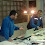42.I think the real inspiration of the "Butterfly Effect" idea is Ray Bradbury's short story "A Sound of Thunder".

43.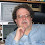And then there is the interesting fact that, from certain points of view, the 2D visualization of the Lorenz attractor looks like a pair of butterfly wings.

1.The Lorentz attractor is a fractal with Hausdorff dimension around 2.06. That is close to two dimensions. However, the chaotic dynamics is tied to the fractional dimension of this attractor set.

44.Sorry for a very naive question, but why do we add (2 1/2)/2 = 1 1/4 days on the second step, and not (5+2 1/2)/2 = 3 3/4 days?

1.Ignat,

Because the additional measurements give you additional prediction time. Also, please avoid submitting a comment multiple times if I am not fast enough approving the first. It doesn't make me any faster, it just clogs up the queue.

45.I always appreciate efforts to set the record straight on popularized terms. For a long time, I pointed people to this article: https://www.livescience.com/17455-butterfly-effect-weather-prediction.html

46.Your explanation of the idea or set of ideas described by the shorthand “Butterfly Effect” is excellent, in my view. I agree entirely—except for the implication that you’re contradicting the version I described in Chaos: Making a New Science. My understanding, based on long discussion with Lorenz, has always been the same as yours. The fact that Lorenz originally used a seagull is also in my book. (The formulation you begin with—that the butterfly may CAUSE a tornado—is a fairly common exaggeration of what I actually wrote.)

I hugely admire Lost in Math, by the way.

47.The butterfly in the Butterfly Effect must be a first cause which is impossible.

48.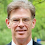I get the sense, matsbergenhov, that you didn't actually read Sabine's post.

sean s.

49.Dear James

As lead author of the paper “The Real Butterfly Effect” to which Sabine refers in her video, could I start by saying that in my opinion your book "Chaos: Making of a New Science" is one of the finest popular science books of all time. It is a masterpiece!

The issue, however, is that Ed Lorenz wrote two key papers on predictability whose mathematical properties are qualitatively different from each other: the famous 1963 paper referred to in your book, and the not-so-famous 1969 paper referred to in Sabine’s video. The 1969 paper was the subject of Lorenz's 1972 AAAS talk about the flap of a butterfly’s wings setting off a tornado in Texas. Lorenz describes this talk in some detail in Appendix 1 of his own 1993 popular book “The Essence of Chaos”.

As Sabine points out, the finite-time loss of predictability Lorenz was describing in his 1969 paper (and in the corresponding 1972 talk) is actually much more radical than the loss of predictability found in the 1963 paper. In the 1963 paper (describing low-order chaos) you can predict as far ahead as you like, providing you know the initial conditions well enough. This is not the case in the model proposed in the 1969 paper where there appears to be an absolute finite-time prediction horizon no matter how well the initial conditions are known. However, the 1969 model is not a rigorous model of fluid flow; indeed, as Sabine says, it is still unknown mathematically whether the Navier-Stokes equations (for fluid motion) have this finite-time predictability property or not.

None of this detracts from your excellent book, of course.

Tim Palmer

50.I tried to make a point, sean s., that there is no such thing as an initial condition.

COMMENTS ON THIS BLOG ARE PERMANENTLY CLOSED. You can join the discussion on Patreon.